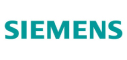New update is available. Click here to update.
Topics

Easy0/40
Average time to solve is 20m+27 more companies

## Problem statement

You are given a singly Linked List of integers. Your task is to return true if the given singly linked list is a palindrome otherwise returns false.

For example:
``````The given linked list is 1 -> 2 -> 3 -> 2-> 1-> NULL.

It is a palindrome linked list because the given linked list has the same order of elements when traversed forwards and backward​.
``````
``````Can you solve the problem in O(N) time complexity and O(1) space complexity iteratively?
``````
Detailed explanation ( Input/output format, Notes, Images )
Constraints :
``````1 <= T <= 10
0 <= L <= 10^5
1 <= data <= 10^9 and data != -1

Where L is the number of nodes in the Linked List.

Time Limit: 1 sec
``````
Sample Input 1 :
``````2
1 2 3 4 5 6 -1
1 2 1 -1
``````
Sample Output 1 :
``````false
true
``````
Explanation for sample 1:
``````For the first test case, it is not a palindrome because Linked List doesn't have the same order of elements when traversed forwards and backwards​.

For the second test case, it is a palindrome linked list because a Linked List has the same order of elements when traversed forwards and backwards​.
``````
Sample Input 2 :
``````2
1 -1
1 10 45 10 1 -1
``````
Sample Output 2 :
``````true
true
``````Console